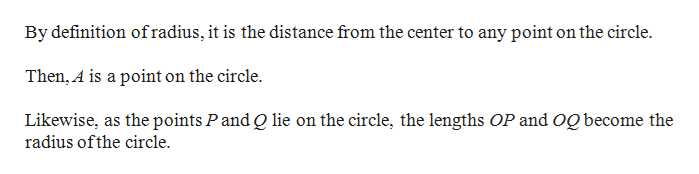Prove the following statement: If P and Q are any points on a circle with center O and radius OA, then OP ∼= OQ.

Question

Prove the following statement: If P and Q are any points on a circle with center O and radius OA, then OP = OQ.

Step 1

Suppose that any two points P and Q lie on the circle centered at O and its radius is OA.

Step 2

From given circle, we have O as center ...help_outlineImage TranscriptioncloseBy definition ofradius, it is the distance from the center to any point on the circle Then, A is a point on the circle. Likewise, as the points Pand Q lie on the circle, the lengths OP and og become the radius ofthe circle fullscreen

Want to see the full answer?

See Solution

Want to see this answer and more?

Our solutions are written by experts, many with advanced degrees, and available 24/7

See Solution
Tagged in

Geometry